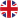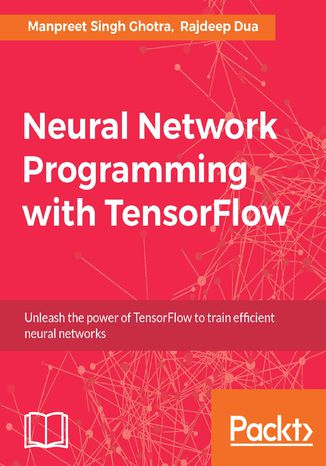# Neural Network Programming with TensorFlow(ebook)(audiobook)(audiobook)Ocena:
Bądź pierwszym, który oceni tę książkę
Stron:
266
3w1 w pakiecie:
PDF
ePub
Mobi

### Ebook

139,00 zł

Przenieś na półkę

Do przechowalni

Neural Networks and their implementation decoded with TensorFlow

## About This Book

• Develop a strong background in neural network programming from scratch, using the popular Tensorflow library.
• Use Tensorflow to implement different kinds of neural networks – from simple feedforward neural networks to multilayered perceptrons, CNNs, RNNs and more.
• A highly practical guide including real-world datasets and use-cases to simplify your understanding of neural networks and their implementation.

## Who This Book Is For

This book is meant for developers with a statistical background who want to work with neural networks. Though we will be using TensorFlow as the underlying library for neural networks, book can be used as a generic resource to bridge the gap between the math and the implementation of deep learning. If you have some understanding of Tensorflow and Python and want to learn what happens at a level lower than the plain API syntax, this book is for you.

## What You Will Learn

• Learn Linear Algebra and mathematics behind neural network.
• Dive deep into Neural networks from the basic to advanced concepts like CNN, RNN Deep Belief Networks, Deep Feedforward Networks.
• Explore Optimization techniques for solving problems like Local minima, Global minima, Saddle points
• Learn through real world examples like Sentiment Analysis.
• Train different types of generative models and explore autoencoders.
• Explore TensorFlow as an example of deep learning implementation.

## In Detail

If you're aware of the buzz surrounding the terms such as "machine learning," "artificial intelligence," or "deep learning," you might know what neural networks are. Ever wondered how they help in solving complex computational problem efficiently, or how to train efficient neural networks? This book will teach you just that.

You will start by getting a quick overview of the popular TensorFlow library and how it is used to train different neural networks. You will get a thorough understanding of the fundamentals and basic math for neural networks and why TensorFlow is a popular choice Then, you will proceed to implement a simple feed forward neural network. Next you will master optimization techniques and algorithms for neural networks using TensorFlow. Further, you will learn to implement some more complex types of neural networks such as convolutional neural networks, recurrent neural networks, and Deep Belief Networks. In the course of the book, you will be working on real-world datasets to get a hands-on understanding of neural network programming. You will also get to train generative models and will learn the applications of autoencoders.

By the end of this book, you will have a fair understanding of how you can leverage the power of TensorFlow to train neural networks of varying complexities, without any hassle. While you are learning about various neural network implementations you will learn the underlying mathematics and linear algebra and how they map to the appropriate TensorFlow constructs.

## Style and Approach

This book is designed to give you just the right number of concepts to back up the examples. With real-world use cases and problems solved, this book is a handy guide for you. Each concept is backed by a generic and real-world problem, followed by a variation, making you independent and able to solve any problem with neural networks. All of the content is demystified by a simple and straightforward approach.

Zamknij